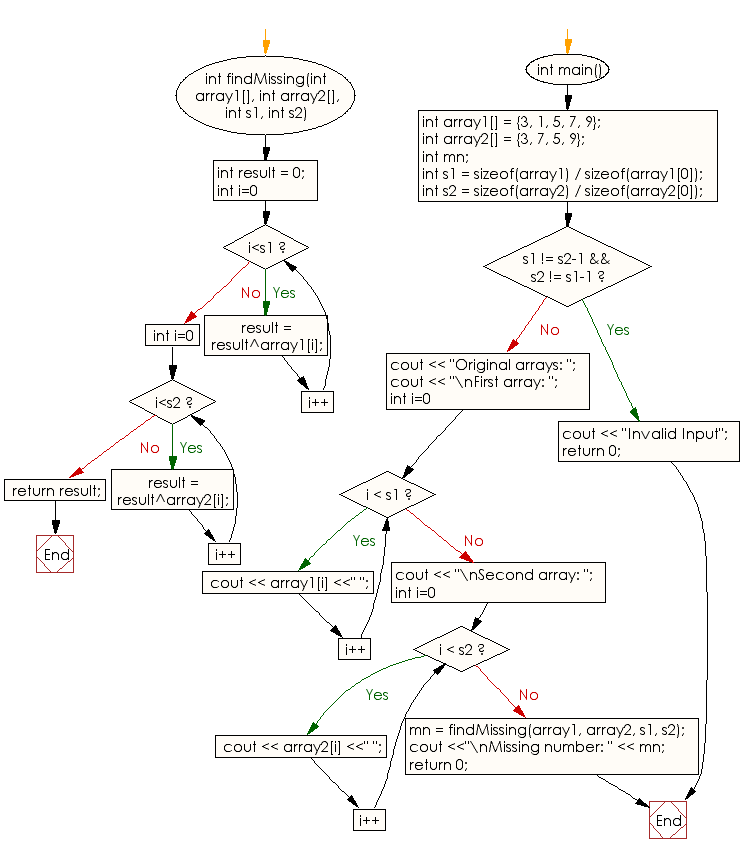﻿ C++ : Find the missing element from two arrays except one# C++ Exercises: Find the missing element from two given arrays of integers except one element

## C++ Array: Exercise-22 with Solution

Write a C++ program to find the missing element from two given arrays of integers except one element.

Sample Solution:

C++ Code :

``````#include <iostream>
using namespace std;

int findMissing(int array1[], int array2[], int s1, int s2)
{
int result = 0;
for (int i=0; i<s1; i++)
result = result^array1[i];
for (int i=0; i<s2; i++)
result = result^array2[i];

return result;
}
int main()
{
int array1[] = {3, 1, 5, 7, 9};
int array2[] = {3, 7, 5, 9};
int mn;
int s1 = sizeof(array1) / sizeof(array1);
int s2 = sizeof(array2) / sizeof(array2);
if (s1 != s2-1 && s2 != s1-1)
{
cout << "Invalid Input";
return 0;
}

cout << "Original arrays: ";

cout << "\nFirst array: ";
for (int i=0; i < s1; i++)
cout << array1[i] <<" ";

cout << "\nSecond array: ";
for (int i=0; i < s2; i++)
cout << array2[i] <<" ";

mn = findMissing(array1, array2, s1, s2);
cout <<"\nMissing number: " << mn;
return 0;
}
``````

Sample Output:

```Original arrays:
First array: 3 1 5 7 9
Second array: 3 7 5 9
Missing number: 1
```

Flowchart:C++ Code Editor:

What is the difficulty level of this exercise?

Test your Programming skills with w3resource's quiz.

﻿